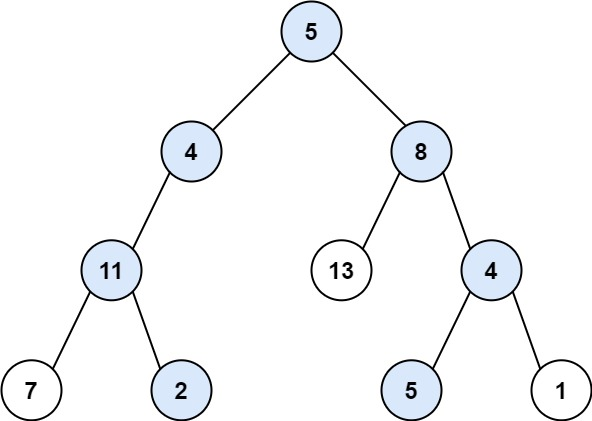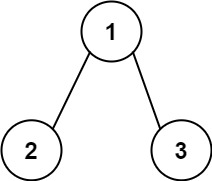113. Path Sum II
Medium
6.9K
139

Given the `root` of a binary tree and an integer `targetSum`, return all root-to-leaf paths where the sum of the node values in the path equals `targetSum`. Each path should be returned as a list of the node values, not node references.

A root-to-leaf path is a path starting from the root and ending at any leaf node. A leaf is a node with no children.

Example 1:```Input: root = [5,4,8,11,null,13,4,7,2,null,null,5,1], targetSum = 22
Output: [[5,4,11,2],[5,8,4,5]]
Explanation: There are two paths whose sum equals targetSum:
5 + 4 + 11 + 2 = 22
5 + 8 + 4 + 5 = 22
```

Example 2:```Input: root = [1,2,3], targetSum = 5
Output: []
```

Example 3:

```Input: root = [1,2], targetSum = 0
Output: []
```

Constraints:

• The number of nodes in the tree is in the range `[0, 5000]`.
• `-1000 <= Node.val <= 1000`
• `-1000 <= targetSum <= 1000`
Accepted
733.7K
Submissions
1.3M
Acceptance Rate
57.1%

Seen this question in a real interview before?
1/4
Yes
No

Discussion (0)

Related Topics